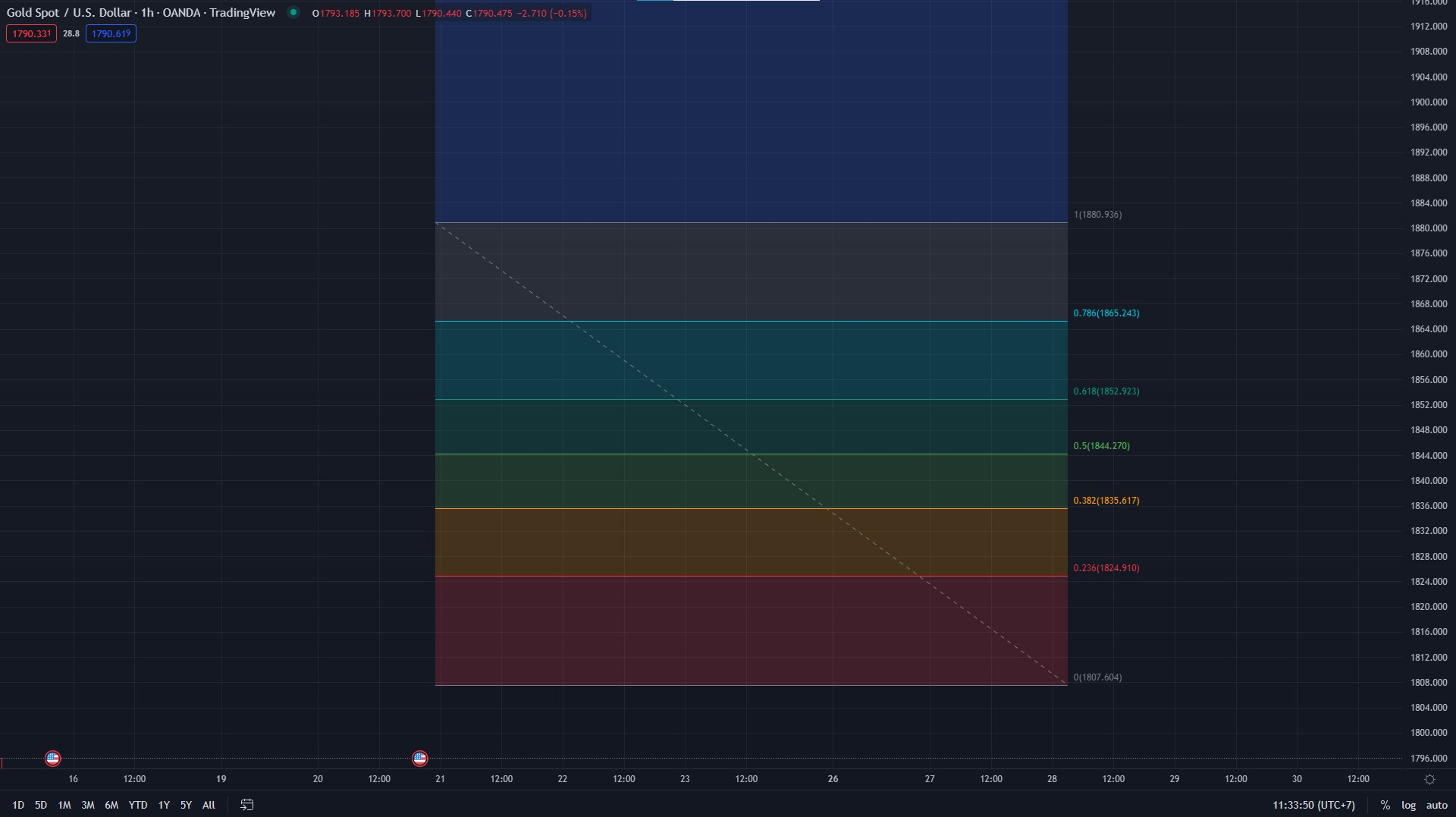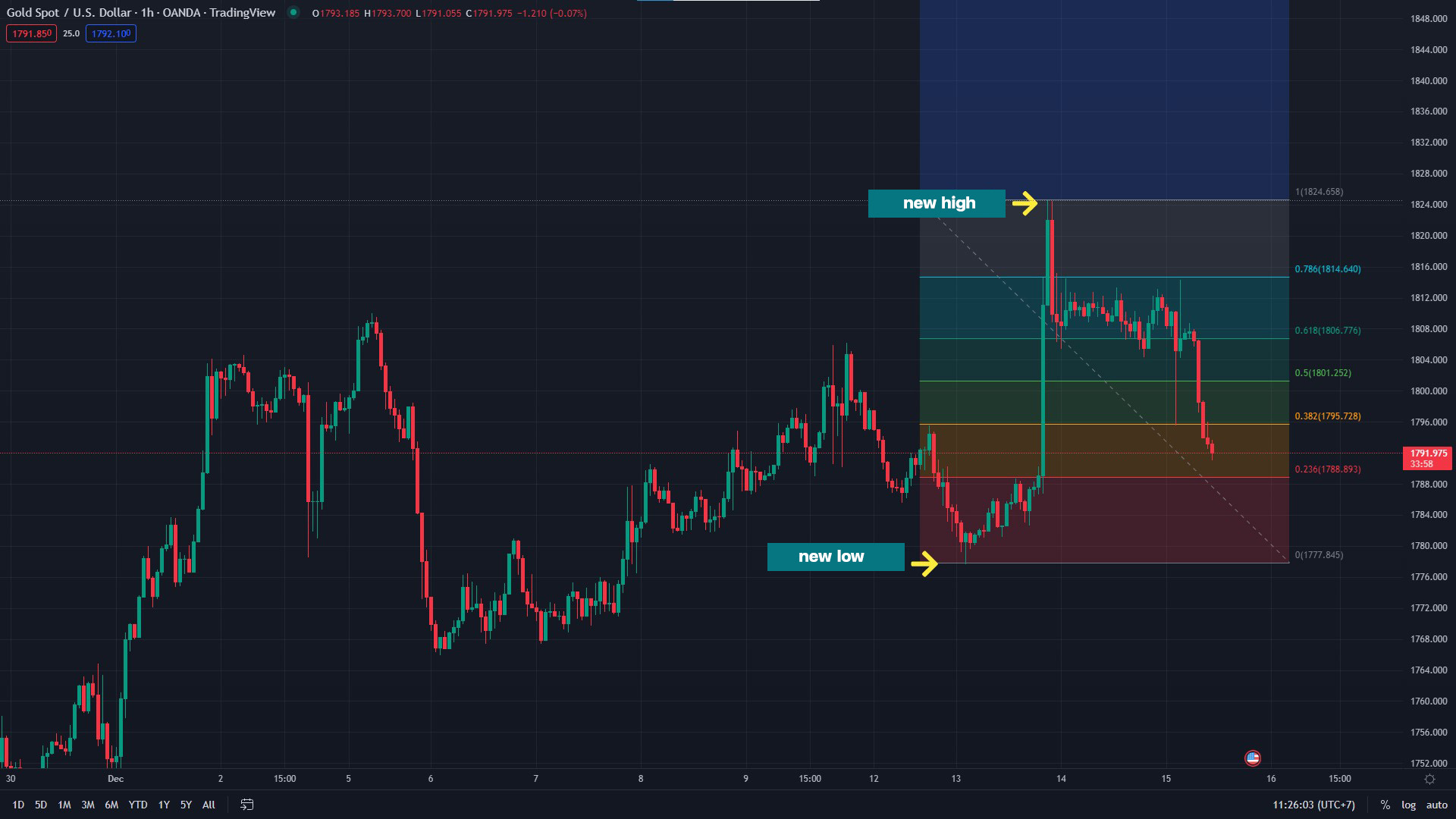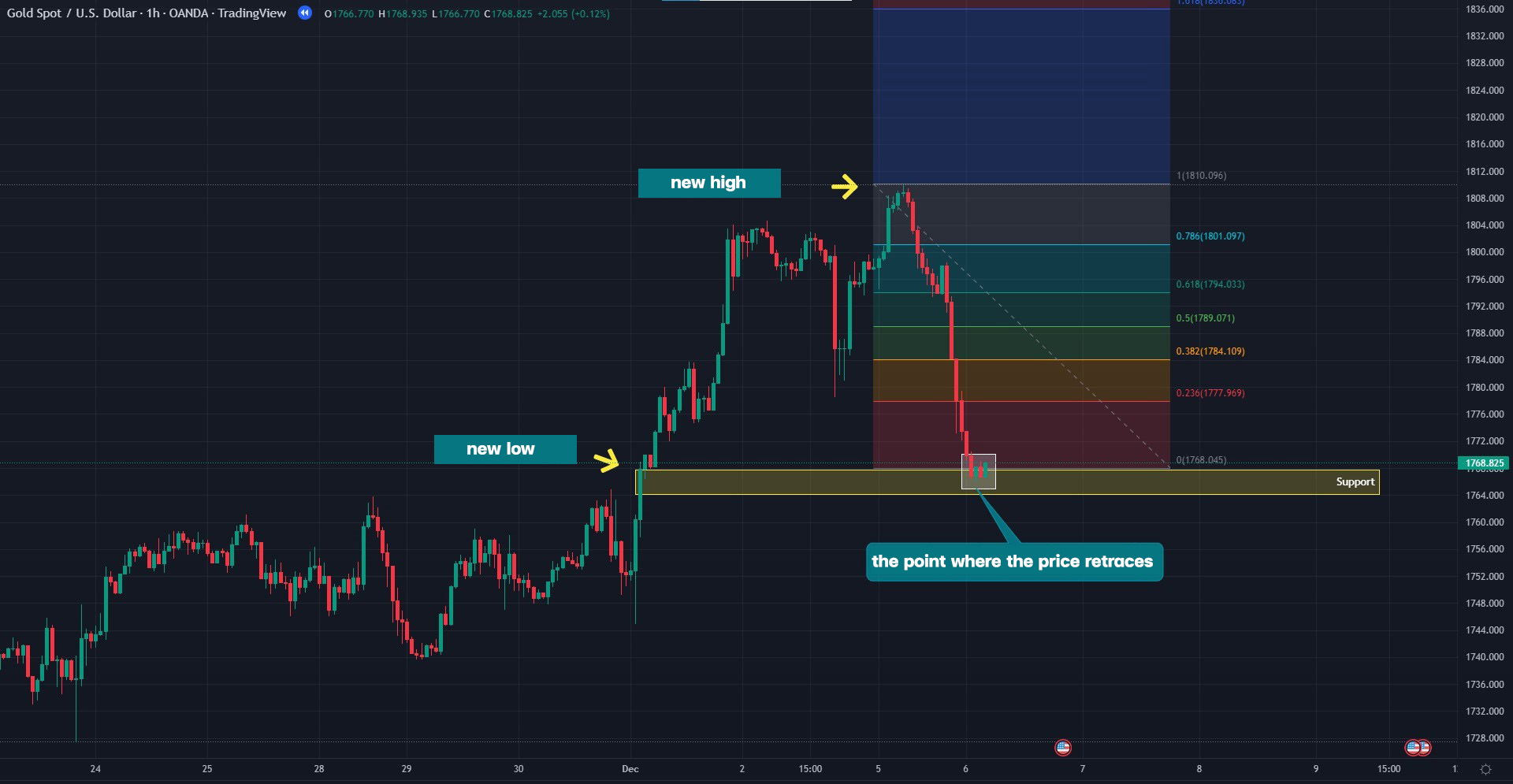# Get to Know Fibonacci Retracement

Fibonacci Retracement, sometimes known as the Golden Ratio, has long been related to the financial industry. It is a popular indicator in all trading markets, especially the Forex market. Fibonacci can effectively identify price reversal points by applying the golden number theory to the graph.

Today, Fxtoday will explain the Fibonacci Retracement, how to use it, and how to apply it to the Forex market.

## What Is Fibonacci Retracement?

Leonardo Fibonacci, an Italian mathematician, invented Fibonacci Retracement as a mathematical tool. He used Fibonacci to identify market reversals by employing the golden ratio and price movements.## How to Correctly Use Fibonacci Retracement

To correctly use Fibonacci retracements, you should keep an eye on the price trend. If the price is rising, you should drag the line from top to bottom and focus on support. However, if the price is falling, you should drag the line from the bottom up and focus on the resistance. Significantly, it must be drawn from one high or low to another. Moreover, Fibonacci Retracement can also be drawn from the past to the present or only in the present.

Nevertheless, traders usually drag in the current price. In addition, you should remember that the longer the Fibonacci is dragged, the longer it takes for the price to reach a meaningful level. Hence, Fibonacci draws can be short or long, depending on the trader's trading strategy.The XAU/USD TF 1h chart sample shows that the chart is in an uptrend. Drawing Fibonacci Retracement from the current high to the recent low is a short draw appropriate for a day trader. If you believe that the graph is about to fall, it may also be depicted as a downtrend.

Each preceding point is crucial since each Fibonacci level is linked with support-resistance. Therefore, trading with Fibonacci Retracement is a trade that focuses on support-resistance points by numerical level of Fibonacci.

Using Fibonacci to trade by drawing support and resistance lines based on the level may determine which level has the most significant reversal. If the price frequently bounces or rests at that level, it suggests that the level is the greatest support-resistance and most probable reversal point.

## Example of Using Fibonacci Retracement in TradingFrom the XAU/USD TF 1h chart, price has taken a pause in that area after the price has declined. As shown in the example, you should drag the Fibonacci Retracement from the current high to the point where the price retraces. In addition, to confirm reversals and breakouts, you should retrace support at Fibonacci level 0.